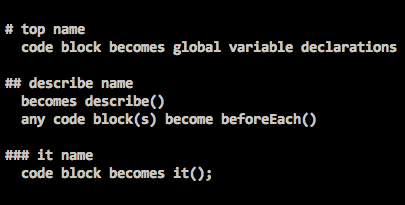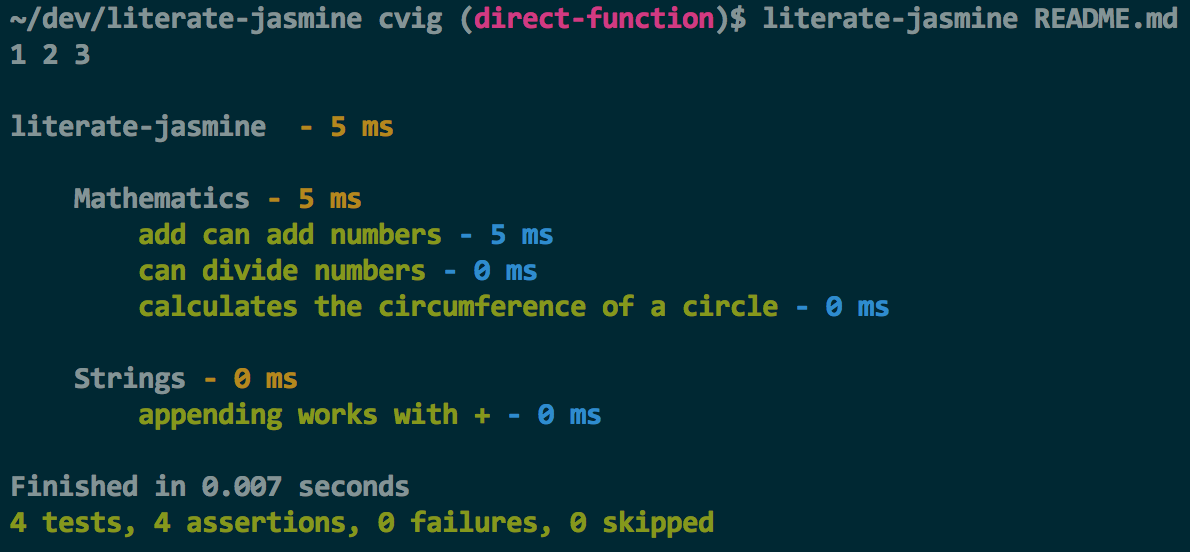Nachos Pillage Milwaukee

# npm

## literate-jasmine

0.1.2 • Public • Published

# literate-jasmine``````var PI;
``````

## Mathematics

``````PI = 22/7;
``````

``````var a = 1,
b = 2;

expect(a + b).toBe(3);
``````

### can divide numbers

``````var a = 6,
b = 2;
``````

And a comment here doesn't break things:

``````expect(a/b).toBe(3);
``````

### calculates the circumference of a circle

Note that we reference the variable PI below that is defined in the code block at the top of this describe (so right under "Mathematics").

``````var circumference = function(radius) {
return 2 * PI * radius;
};

expect(circumference(5)).toBe(2 * 22/7 * 5);
``````

## Strings

### appending works with +

``````var text = "abc";

expect(text + "d").toBe("abcd");
``````

## Asynchronous

### works with done

``````setTimeout(function() {
expect(true).toBe(true);
done();
}, 50);
``````

The file you are currently reading has a markdown structure (which includes the main header above and the other parts below) that is parsed into a tree:

• literate-jasmine
• Mathematices
• calculates the circumference of a circle
• Strings
• appending works with +
• Asynchronous
• works with done

Which is then uses the Jasmine `describe`, `it` and `beforeEach` to setup the tests and then run them.

Note that there is a convention -- a hard rule that there will be a top header (top describe), one or more headers below that (describes) and one or more header below each of those (it blocks).*This hard limitation makes sense:

• forces documentation to be simple
• nested describes indicate that extracted some code to helper functions is probably a sensible solution instead of adding more nesting
• easier to parse both as a human and as a computer
• keeps everything simple

`literate-jasmine README.md`(If you're working on this project, run `./bin/literate-jasmine` instead.)

Take a close look at how scope works for globals. In the Mathematics section below, we reference `PI` to reset it as a `beforeEach` so every test has `PI` reset to the correct value. The actual declaration of `PI` as a variable happens on the fourth line of this README. The root describe treats any code blocks after it as global setup.

## Keywords

none

### Install

`npm i literate-jasmine`

### Repository

github.com/cymen/literate-jasmine

### Homepage

github.com/cymen/literate-jasmine